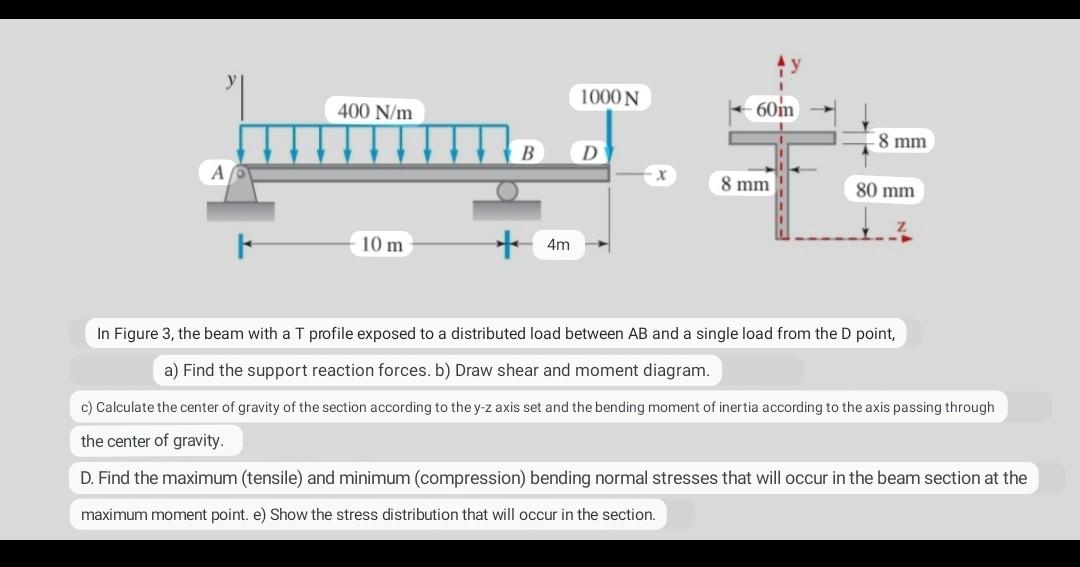# (Solved):   In Figure 3, the beam with a T profile exposed to a distributed load between \( A B \) and ...In Figure 3, the beam with a T profile exposed to a distributed load between \( A B \) and a single load from the \( D \) point, a) Find the support reaction forces. b) Draw shear and moment diagram. c) Calculate the center of gravity of the section according to the \( y \)-z axis set and the bending moment of inertia according to the axis passing through the center of gravity. D. Find the maximum (tensile) and minimum (compression) bending normal stresses that will occur in the beam section at the maximum moment point. e) Show the stress distribution that will occur in the section.

We have an Answer from Expert